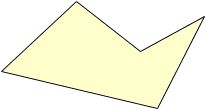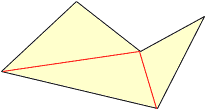Sender: melanie Subject: area of shapes what is the formula to calculate the area of different shapes? Hi Melanie, There is not one formula for the area of different shapes. The best you can do is to divide the shape into pieces where you can find the areas of the individual pieces. If the shape is a polygon, that is the boundary is made of straight line segments, then you can always divide it into triangles. For example the area of the shapecan be found by dividing it into three trianglesand adding the areas of the three triangles.   For some familiar polygons; squares, rectangls, parallelograms, trapezoids, and triangles there is a Universal Area Formula. Cheers, Harley Go to Math Central